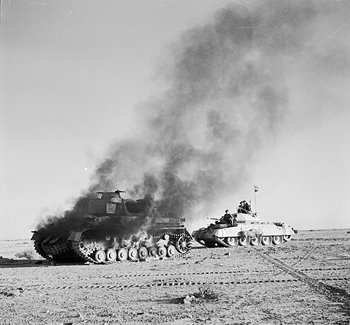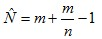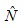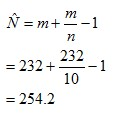# The German Tank Problem

Statistical Analysis Techniques, WarDuring World War 2, the Western Allies used a simple formula to estimate the rate at which German tanks were being produced, based on the serial numbers obtained from captured and destroyed tanks.

The formula is the following:whereis estimated total number of objects (e.g. German tanks)
m  is the highest sampled serial number
n  is the sample size (e.g. the number of captured/destroyed German tanks)

For example, let’s say 10 tanks were captured/destroyed, and the following serial numbers were obtained:
117, 232, 122, 173, 167, 12, 168, 204, 4, 229

The highest serial number obtained was 232, therefore m = 232.It so happens that these 10 serial numbers were drawn randomly from a (rounded) uniform distribution with minimum 1, and maximum 255.

How well the formula performed

The formula performed much better than the conventional intelligence estimates.  Conventional intelligence estimates were based on counting the number of tanks on the battlefield and by secretly observing factories.

Through conventional intelligence it was estimated that the Germans were producing around 1400 tanks per month, from June 1940 to September 1942.  The statistical estimate was 246 tanks per month.  After the war, German production figures showed the actual number to be 245.

Estimates for some specific months:

 Month Statistical Estimate Intelligence Estimate German Records June 1940 169 1000 122 June 1941 244 1550 271 August 1942 327 1550 342

The statistical estimates were useful because they gave the Allies an idea of whether or not an attack on the western front could succeed.

Other applications

This formula can be applied to other things with serial numbers.  For example, with serial numbers gathered through online discussions, the same formula was used to estimate the number of iphones sold.  It was estimated that Apple had sold around 9.1 million phones to the end of September 2008.